# Differential equations Questions and Answers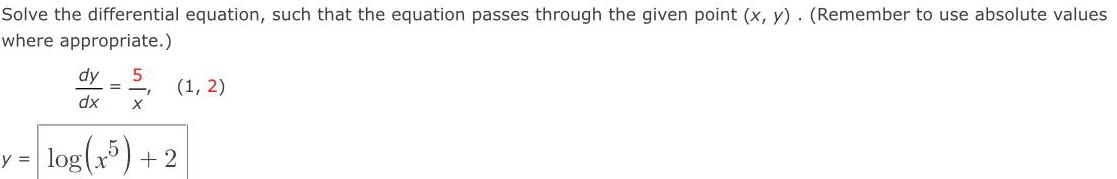Math
Differential equations
Solve the differential equation, such that the equation passes through the given point (x, y). (Remember to use absolute values where appropriate.) dy/dx = 5/x (1, 2) y = log(x5) +2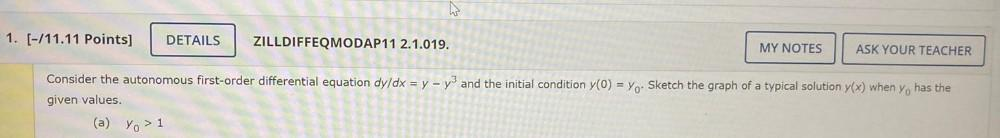Math
Differential equations
Consider the autonomous first-order differential equation dy/dx = y - y³ and the initial condition y(0) = y₀. Sketch the graph of a typical solution y(x) when y₀, has the given values. (a) y₀ > 1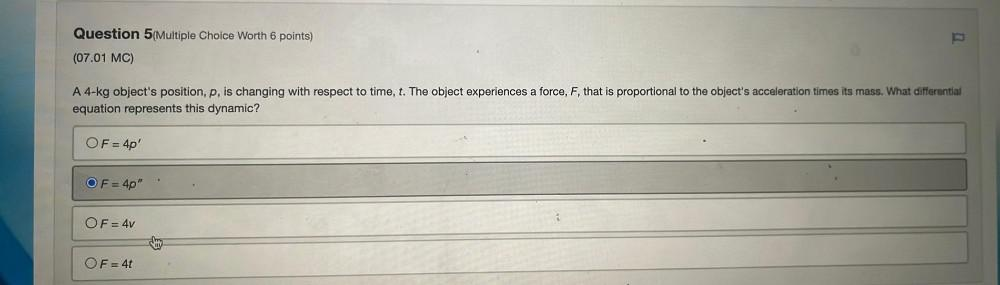Math
Differential equations
A 4-kg object's position, p, is changing with respect to time, t. The object experiences a force, F, that is proportional to the object's acceleration times its mass. What differential equation represents this dynamic? A. F = 4p' B. F = 4p" C. F = 4v D. F = 4t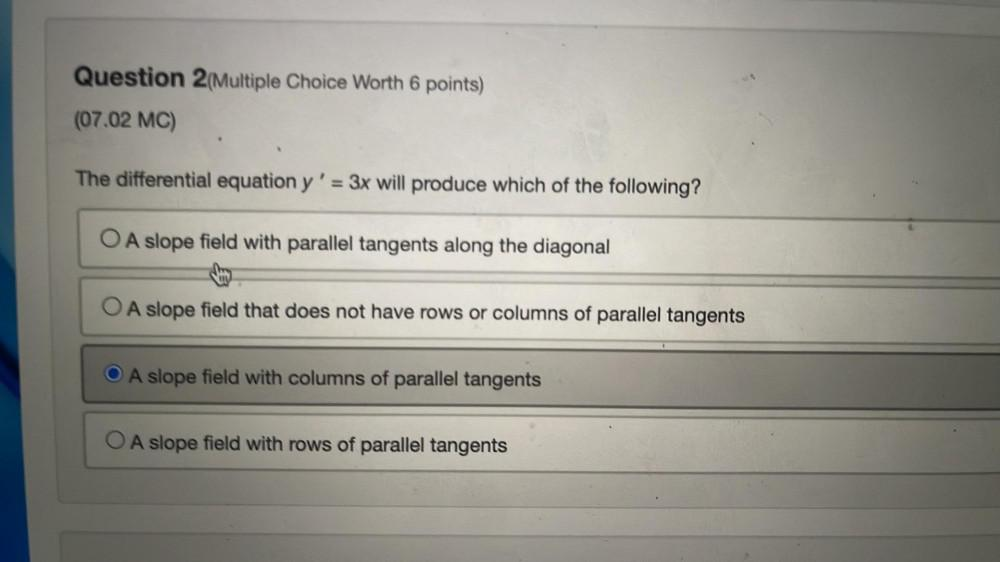Math
Differential equations
The differential equation y' = 3x will produce which of the following? A. A slope field with parallel tangents along the diagonal B. A slope field that does not have rows or columns of parallel tangents C. A slope field with columns of parallel tangents D. A slope field with rows of parallel tangents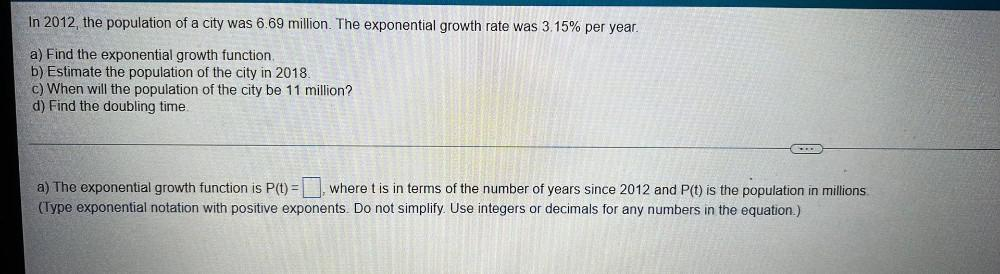Math
Differential equations
In 2012, the population of a city was 6.69 million. The exponential growth rate was 3.15% per year. a) Find the exponential growth function. b) Estimate the population of the city in 2018. c) When will the population of the city be 11 million? d) Find the doubling time. a) The exponential growth function is P(t) = ▢ where t is in terms of the number of years since 2012 and P(t) is the population in millions. (Type exponential notation with positive exponents. Do not simplify. Use integers or decimals for any numbers in the equation.)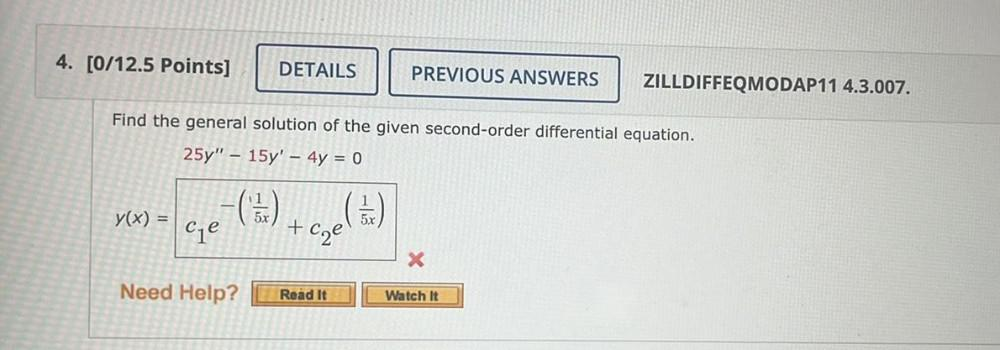Math
Differential equations
Find the general solution of the given second-order differential equation. 25y" – 15y' - 4y = 0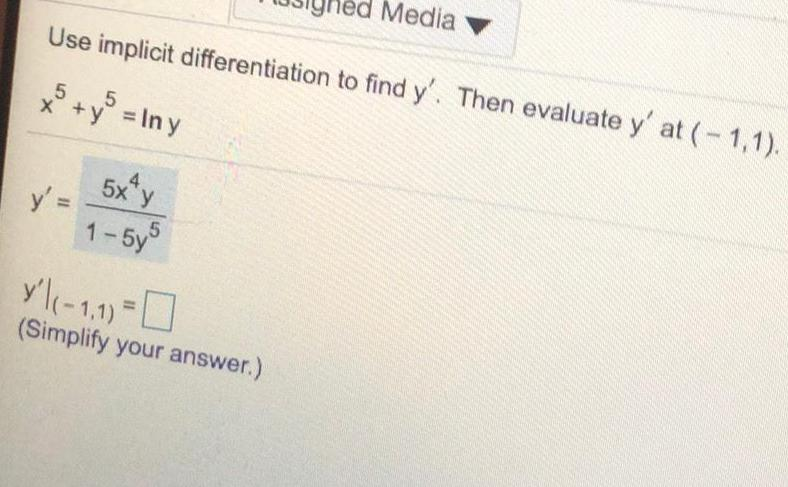Math
Differential equations
Use implicit differentiation to find y'. Then evaluate y' at (-1,1). x^5 + y^5 = lny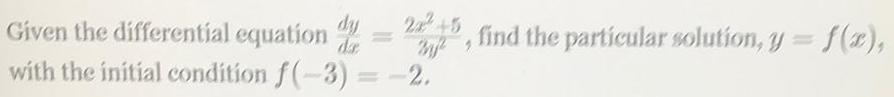Math
Differential equations
Given the differential equation dy/dx = 2x^2+5/3y^2, find the particular solution, y = f(x), with the initial condition f(-3) = -2.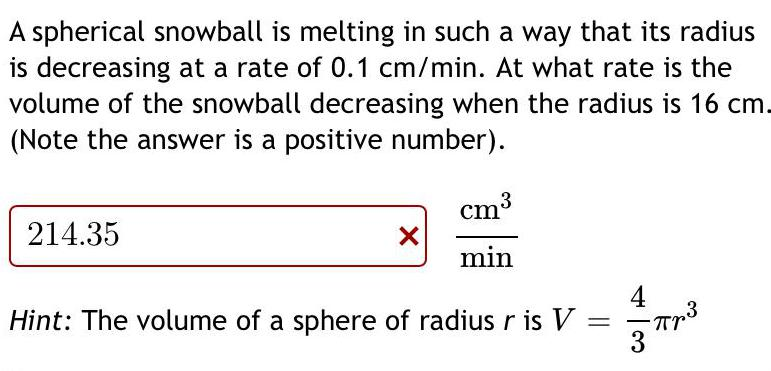Math
Differential equations
A spherical snowball is melting in such a way that its radius is decreasing at a rate of 0.1 cm/min. At what rate is the volume of the snowball decreasing when the radius is 16 cm. (Note the answer is a positive number). cm³ min Hint: The volume of a sphere of radius r is V = 4/3πr ³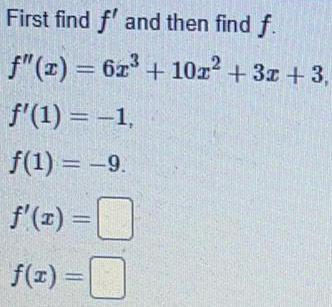Math
Differential equations
First find f' and then find f. f"(x) = 6x³ + 10x² + 3x +3, f'(1) = -1, f(1) = -9. f'(x) = f(x) =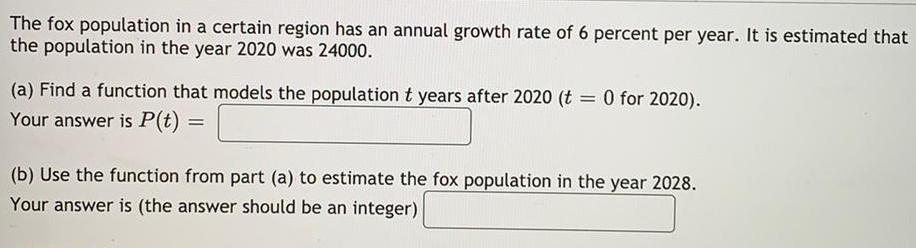Math
Differential equations
The fox population in a certain region has an annual growth rate of 6 percent per year. It is estimated that the population in the year 2020 was 24000. (a) Find a function that models the population t years after 2020 (t = 0 for 2020). Your answer is P(t) (b) Use the function from part (a) to estimate the fox population in the year 2028. Your answer is (the answer should be an integer)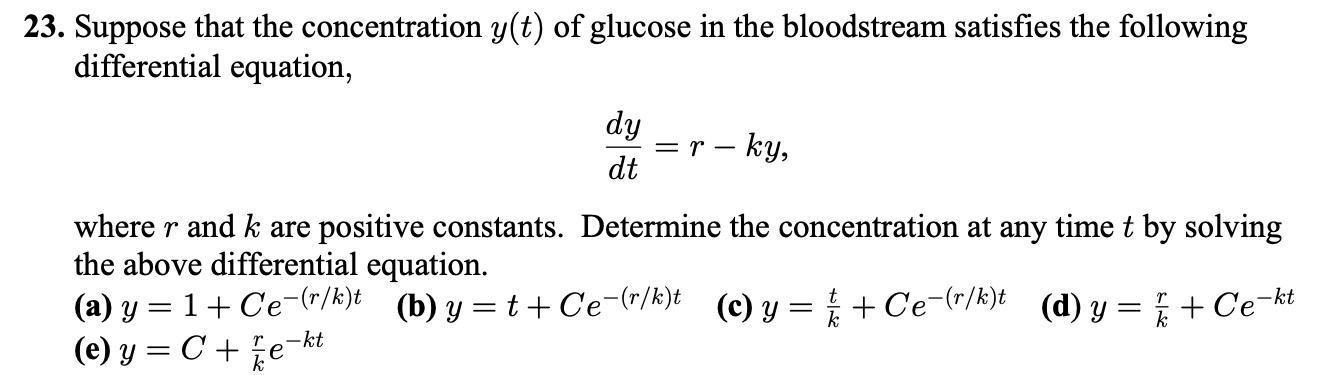Math
Differential equations
Suppose that the concentration y(t) of glucose in the bloodstream satisfies the following differential equation, dy/dt =r-ky, where r and k are positive constants. Determine the concentration at any time t by solving the above differential equation. (a) y = 1+ Ce(r/k)t (b) y=t+Ce-(r/k)t (c) y = // + Ce(r/k)t (d) y = / + Ce-kt (e) y = C + e-kt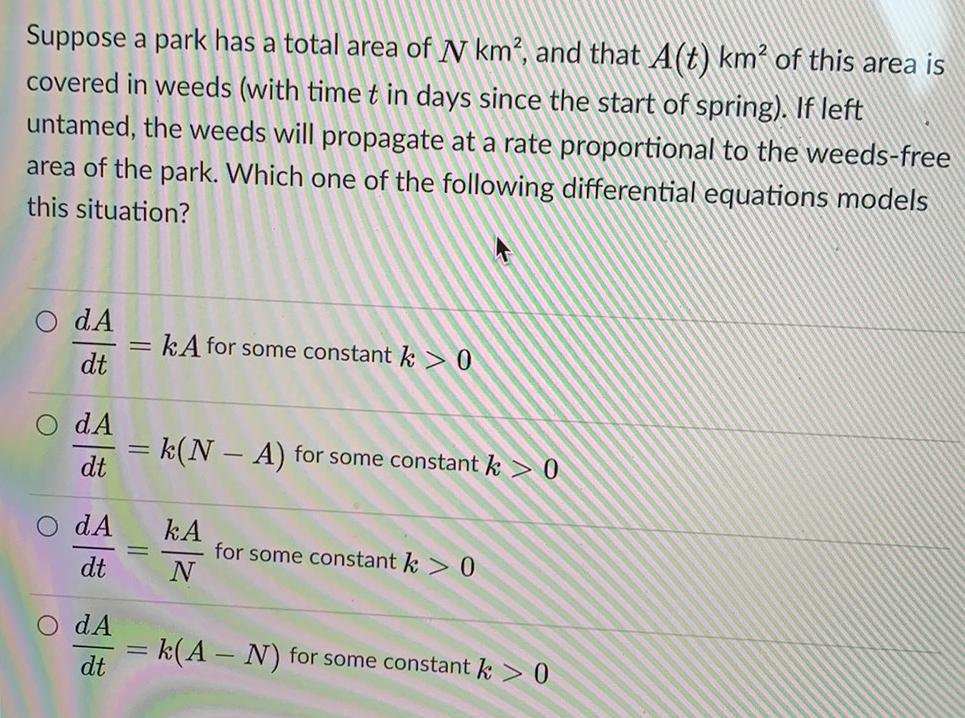Math
Differential equations
Suppose a park has a total area of N km², and that A(t) km² of this area is covered in weeds (with time t in days since the start of spring). If left untamed, the weeds will propagate at a rate proportional to the weeds-free area of the park. Which one of the following differential equations models this situation?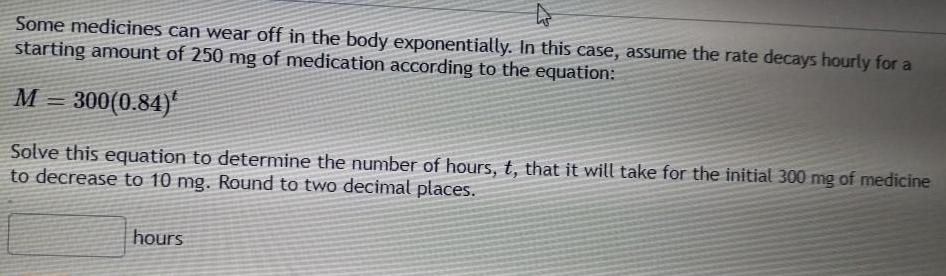Math
Differential equations
Some medicines can wear off in the body exponentially. In this case, assume the rate decays hourly for a starting amount of 250 mg of medication according to the equation: M = 300(0.84)t Solve this equation to determine the number of hours, t, that it will take for the initial 300 mg of medicine to decrease to 10 mg. Round to two decimal places.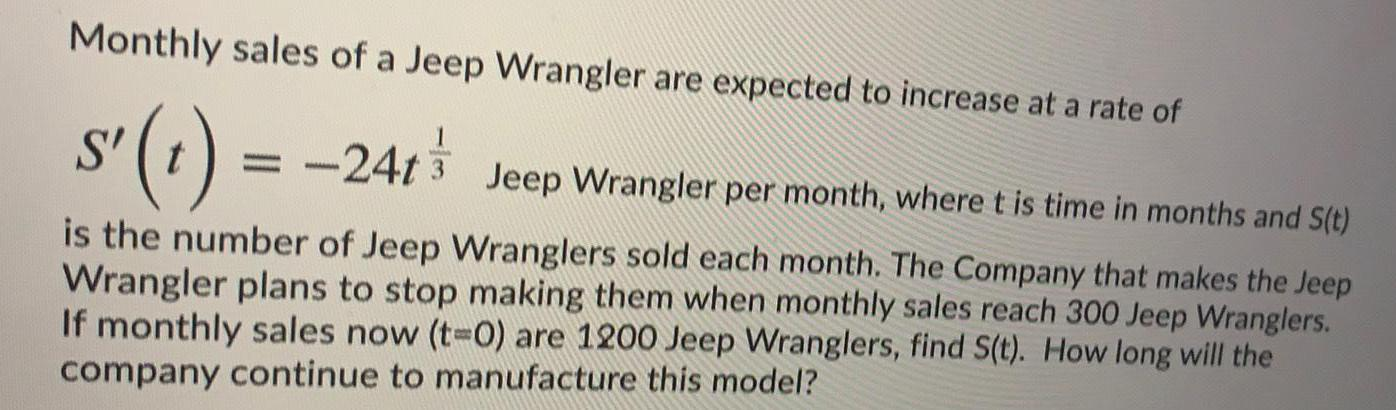Math
Differential equations
Monthly sales of a Jeep Wrangler are expected to increase at a rate of S' (t) = -24t3 Jeep Wrangler per month, where t is time in months and S(t) is the number of Jeep Wranglers sold each month. The Company that makes the Jeep Wrangler plans to stop making them when monthly sales reach 300 Jeep Wranglers. If monthly sales now (t-0) are 1200 Jeep Wranglers, find S(t). How long will the company continue to manufacture this model?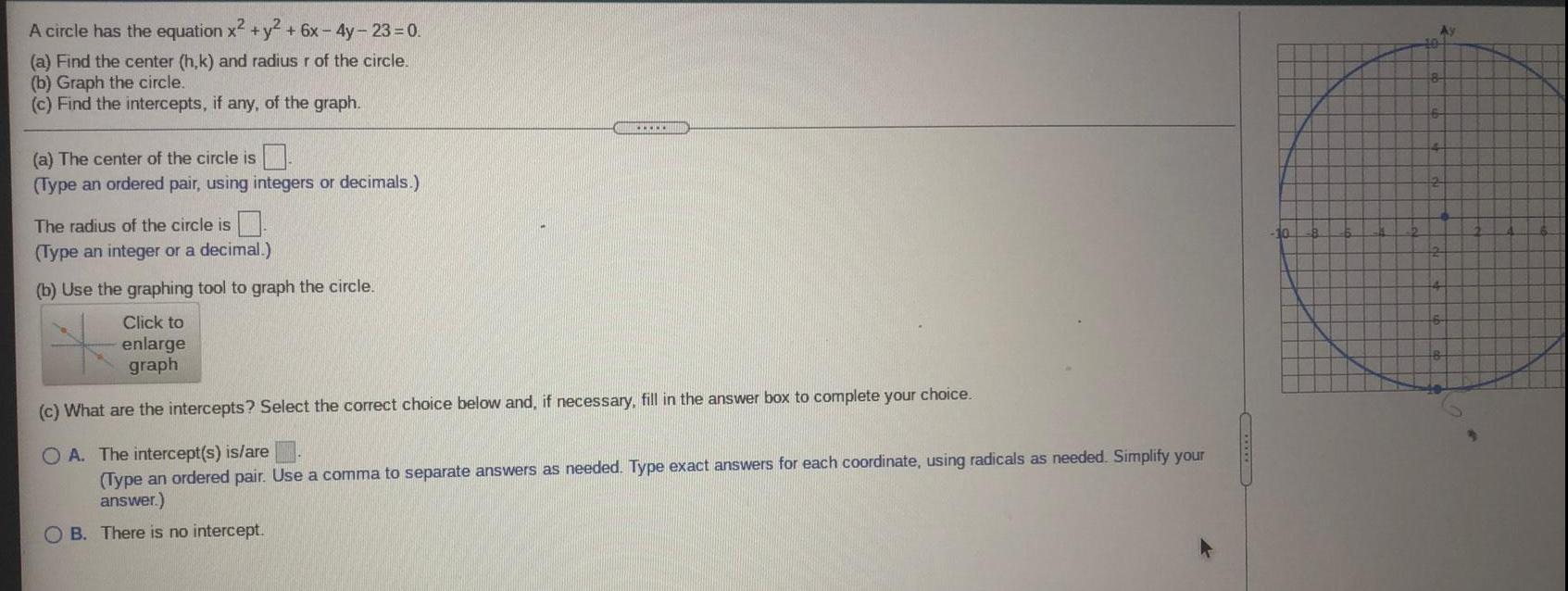Math
Differential equations
A circle has the equation x2 + y² + 6x-4y-23=0. (a) Find the center (h,k) and radius r of the circle. (b) Graph the circle. (c) Find the intercepts, if any, of the graph. (a) The center of the circle is (Type an ordered pair, using integers or decimals.) The radius of the circle is (Type an integer or a decimal.) (b) Use the graphing tool to graph the circle. (c) What are the intercepts? Select the correct choice below and, if necessary, fill in the answer box to complete your choice. A. The intercept(s) is/are (Type an ordered pair. Use a comma to separate answers as needed. Type exact answers for each coordinate, using radicals as needed. Simplify your answer.) B. There is no intercept.